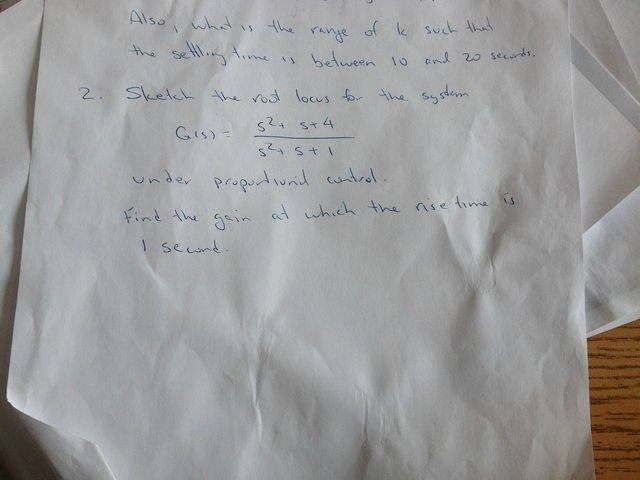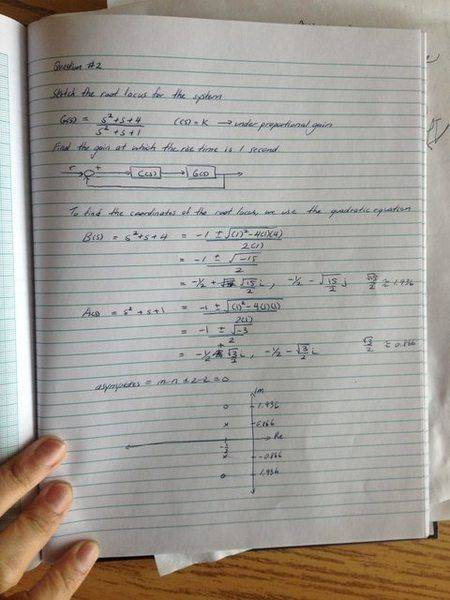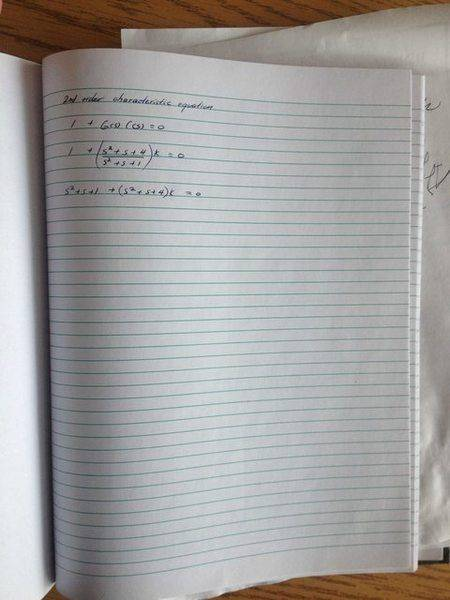# Control Systems - Root Locus with proportional control

xopher

## Homework StatementSee below

## The Attempt at a Solution

Root Locus? Easy Peasy right? Based on provided equation G(s), i solved the coordinates of the root locus diagram using quadratic equation.

For the 2nd part of the question, i have to find gain at which rise time is 1 second. With previous sample questions, we would use s^2 + 2zWs + W^2 for the 2nd order denominator to finish the question based on given parameters (ie dampening ratio, percentage overshoot etc). How do i deal with the numerator?Gold Member
I cannot remember root locus at all. sorry

that being said MATLAB will do a root locus plot for you
you can use that to check your work. I haven't done root locus by hand since I was required too in school.

note: Make sure you understand the material for your tests. for now only use MATLAB as a tool to help you check your answer. Do not just copy the graph to a piece of paper.

http://www.mathworks.com/help/control/ref/rlocus.html

xopher
I actually just figured it out!. If you create a transfer function using G and C, you can easily match it up to the characteristic equation for 2nd order systems and solve accordingly. I double checked this on MATLAB so i know its the correct approach.